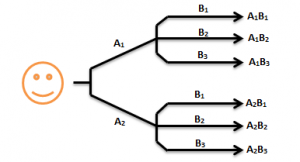Checkout JEE MAINS 2022 Question Paper Analysis : Checkout JEE MAINS 2022 Question Paper Analysis :

# Fundamental Principle Of Counting

On a bright sunny day, you go for shopping. You select a nice pair of jeans and decide to pay using your credit card. But you suddenly realize that you are not able to recollect your pin. What a tragedy!! Now all you can think of is to list all the possible combinations to figure out your pin. How many possible combinations can you make? The answer to this question is difficult if we keep listing each possible combination and counting. In situations like these, the fundamental principle of counting or the multiplication principle comes to our rescue. Let us see what the fundamental principle of counting is all about.

## Fundamental Counting Principle Notes

Suppose you have 2 pairs of shoes and 3 pairs of socks. In how many ways can you wear them? Now, the possible ways of choosing a pair of shoes are 2, since 2 pairs of shoes are available. With any pair of shoes, any of the 3 pairs of socks can be worn at a time. Therefore, for each pair of shoes, there are 3 choices of socks. Similarly, with 2 pairs of shoes, there are 6 choices of socks available since 2 × 3 = 6. This can be understood more clearly with the help of the following figure. Let A1 and A2 represent the 2pairs of shoes and B1, B2, B3 represent the 3pairs of socks.In the problem stated above, we use the fundamental principle of counting to get the result. The multiplication principle states that if an event A can occur in x different ways and another event B can occur in y different ways then there are x × y ways of occurrence of both the events simultaneously.

This principle can be used to predict the number of ways of occurrence of any number of finite events. For example, if there are 4 events which can occur in p, q, r and s ways, then there are p × q × r × s ways in which these events can occur simultaneously.

Well, the answer to the initial problem statement must be quite clear to you by now. The credit card pin would involve a sequence of 4 digits and each digit can vary between 0 – 9. Thus, the number of ways of occurrence of each digit is 10. Since it’s a 4 digit pin, the number of possible combinations is 10 × 10 × 10 × 10 = 10000. There are 10000 combinations possible out of which 1 is correct. Well, good luck trying to figure that out. This explains us the fundamental principle of counting which lays the foundation for permutations and combinations.

Dependent or Independent?

The fundamental principle of counting only works when the choice that is to be made are independent of each other.

If one possibility depends on another then a simple multiplication does not work.